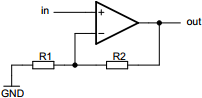# Non-inverting operational amplifier calculator

## Calculates properties of inverting operational amplifier circuit

 Example 1: Must calculate the resistance of R2 to have output of 70 millivolts at 800 microvolt input and R1 value of 10 kilo-ohms View example
 Input voltage volts Output voltage volts Resistor R1 ohms Resistor R2 ohmsYou may use one of the following SI prefix after a value: p=pico, n=nano, u=micro, m=milli, k=kilo, M=mega, G=giga

 Results Resistor R2 ~865 kilo-ohms Voltage gain 87.5 Amplification ~38.84 dB